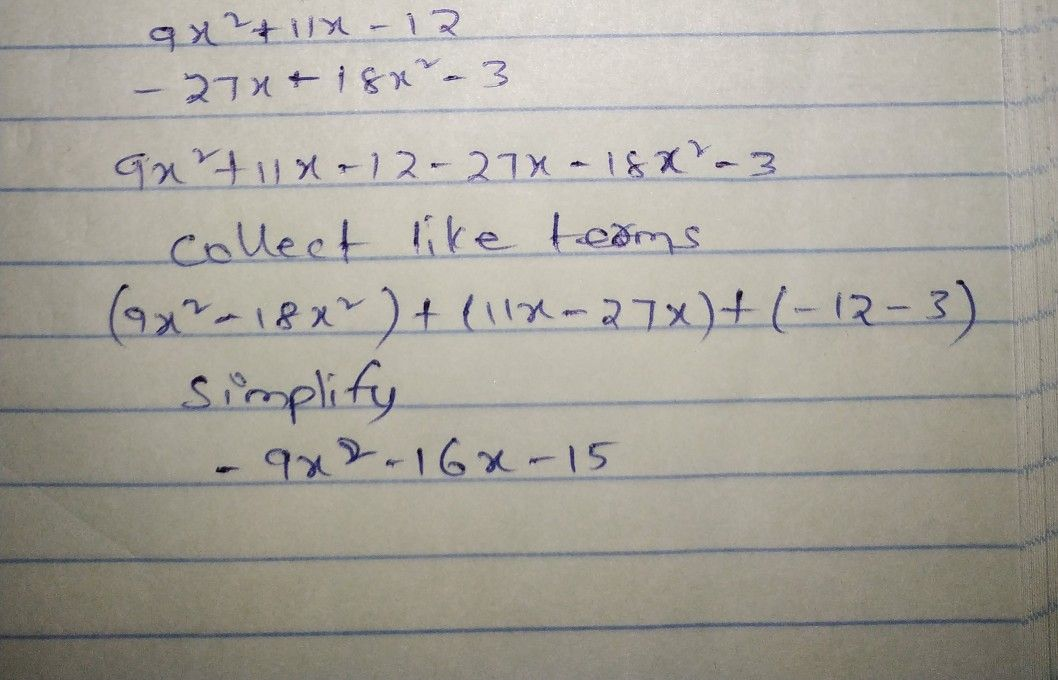Symbol
Problem$2$ $9x-+11x=18$ $-18x^{2-3}$ $=$ $8i+x-18x^{2}-3$
10th-13th grade
Other
SolutionQanda teacher - SahilRawat